# PSAT Math : How to find the length of an edge of a cube

## Example Questions

### Example Question #2 : Solid Geometry

The number of square units in the surface area of a cube is twice as large as the number of cubic units in its volume. What is the cube's volume, in cubic units?

108

216

9

27

36

27

Explanation:

The number of square units in the surface area of a cube is given by the formula 6s2, where s is the length of the side of the cube in units. Moreover, the number of cubic units in a cube's volume is equal to s3

Since the number of square units in the surface area is twice as large as the cubic units of the volume, we can write the following equation to solve for s:

6s2 = 2s3

Subtract 6s2 from both sides.

2s3 – 6s2 = 0

Factor out 2s2 from both terms.

2s2(s – 3) = 0

We must set each factor equal to zero.

2s2 = 0, only if s = 0; however, no cube has a side length of zero, so s can't be zero.

Set the other factor, s – 3, equal to zero.

s – 3 = 0

s = 3

This means that the side length of the cube is 3 units. The volume, which we previously stated was equal to s3, must then be 33, or 27 cubic units.

### Example Question #1 : How To Find The Length Of An Edge Of A Cube

What is the surface area of a cube whose volume is 512 cubic feet?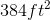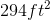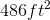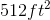Explanation:

In order to find the surface area of a cube, we need to solve for the length of each side,.

Recall the formula for volume: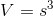Plug in what we know and solve for: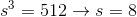Now plug this value into the surface area formula: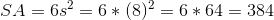### Example Question #2 : How To Find The Length Of An Edge Of A Cube

If the volume of a cube is 64 cubic inches, then it has an edge length of _______.

4 in

2 in

9 in

8 in

6 in

4 in

Explanation: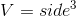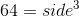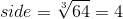### Example Question #3 : How To Find The Length Of An Edge Of A Cube

If the volume of a cube is 512 units, what is the length of one edge of the cube?Explanation:

The volume of a cube is length x width x height. Since it's a cube, though, the length, width, and height are all equal, and equivalent to the length of one edge of the cube.  Therefore, to find the lenght of an edge of the cube, just find the cube root of the volume. In this case, the cube root of 512 is equal to 8.

### Example Question #4 : How To Find The Length Of An Edge Of A Cube

Find the length of an edge of a cube that has a volume of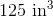.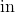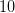Explanation:

All the edges of a cube have the same length, and the volume of a cube is the length of an edge taken to the third power.

So if we take the edge of the cube to be of length x, then: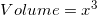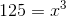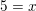So the length of the edge of a cube with a volume of 125 is 5.

### Example Question #5 : How To Find The Length Of An Edge Of A Cube

A certain shipping company has cubic boxes. One of these boxes has a volume of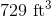. How long are each of the sides of the box in feet?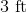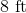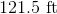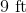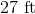Explanation:

The formula for the volume of a cube iswhereis the length of a side.

Here, the volume is 729. To find the side length, take the cube root of both sides: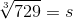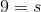The cube root of 729 is 9, so the length of each side of the cube is 9 feet.

### Example Question #6 : How To Find The Length Of An Edge Of A Cube

If a cube has a volume of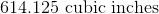cubic inches, approximately how long, in feet, is one edge of the cube?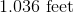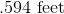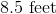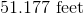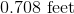Explanation:

The formula for the volume of a cube is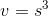where s is any edge.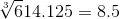This means one edge of the cube is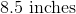. We then divide 8.5 by 12 to convert to feet.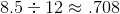feet.